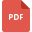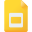# expressions tutorialsSimplifying Algebraic Expressions Multiplying and Dividing
Multiplication, Grade 8, United States, DivisionAlgebraic Expressions and Number Sentences
Algebra, Grade 3, United States, Expressions, Number SentencesMathematics, High School, United States, Radical ExpressionsMathematics, High School, United States, Radical ExpressionsExpanding Algebraic Expressions: Multiplication
Multiplication, Grade 8, United States, MathematicsSolving Equations containing Binomial Expressions
Binomial Theorem, Grade 8, United States, Mathematics27 - Introducing to Algebraic Expressions
Algebra, Grade 8, United States, MathematicsSimplifying Algebraic Expressions
Mathematics, Grade 7, United States, AlgebraExpressions and Equations
Expressions, Grade 4, United States, Linear EquationsOrder of Operations to Evaluate Expressions
Order of Operations, Grade 3, United States, MathematicsSubstitution into Algebraic Expressions
Mathematics, Grade 8, United States, AlgebraCombining and Simplifying Algebraic Expressions
Algebra, Grade 8, United States, MathematicsAlgebraic Expressions Practice Worksheet
Mathematics, Grade 8, United States, AlgebraLearn Algebraic Expression Simplification
Expressions, Grade 3, United States, AlgebraEquations with Rational Expressions and Graphs
Rational Functions, High School, United States, MathematicsAlgebraic expressions
Mathematics, Grade 7, United States, AlgebraRadical Expressions, High School, United States, MathematicsEvaluating Expressions
Mathematics, Grade 7, United States, ExpressionsRational Expressions and Functions; Multiplying and Dividing
Rational Functions, High School, United States, MathematicsExpanding and simplifying algebraic expressions
Mathematics, Grade 7, United States, AlgebraRational Expressions, Vertical Asymptotes, and Holes
Rational Functions, High School, United States, MathematicsOperations with Algebraic Expressions
Mathematics, Grade 7, United States, AlgebraLearn How to Write Numbers in Expanded Form
Expressions, Grade 3, United States, Number SystemSimplification of Algebraic Expressions
Algebra, Grade 8, United States, Mathematics